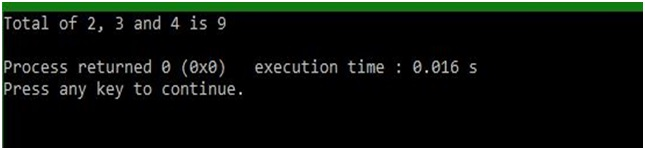# va_start() and va_end() functions of stdarg.h in C

In this article, we are going to learn about the va_start() and va_end() functions of stdarg.h header file in C programming language and use it to initializes the variable argument list referred to by ap.
Submitted by Abhishek Sharma, on April 20, 2018

These are helpful function which tells us about the arguments which are being passed to the function. There are lots of cases where you don’t want to make different functions for the different numbers of argument.

For example, you have to make two functions for these things (if you are not using an array).

```    add(int a, int b) {
return a + b;
}

add(int a, int b, int c) {
return a + b +c;
}
```

Now you can make a single function like this:

```    add(int I, ...) {
va_list L;
int z=0;
va_start(L, a);
for (int i=0; i < a; i++)
z = z + va_arg(L, int);
va_end(L);
}
```

Well, simple way is to use array.

## stdarg.h- va_start() and va_end() functions Example in C

﻿

```#include <stdio.h>
#include <stdarg.h>

int fun(int a, ...)
{
// define type of variable
va_list L;
int z;

z = 0;

va_start(L, a);

// Loop to adding the int values
for (int i=0; i < a; i++)
{
z = z + va_arg(L, int);
}

va_end(L);

return z;
}

int main()
{
// Define temporary variables
int x, y, z;
int k;

x = 2;
y = 3;
z = 4;

// calling function
k = fun(3, x, y, z);

// displaying message with result
printf("Total of %d, %d and %d is %d\n", x, y, z, k);

return 0;
}
```

Output